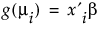For situations such as these that fall into a wider range of data analysis problems, generalized linear models can be applied. Generalized linear models are an extension of traditional linear models. A generalized linear model consists of a linear component, a link function, and a variance function. The link function,, is a monotonic and differentiable function that describes how the expected value of Yi is related to the linear predictors. An example of generalized linear regression is Poisson regression, whereis the link function. For a complete list of the generalized linear regression models available using the Generalized Linear Models personality of the Fit Model platform, see Statistical Details.
An important aspect of fitting generalized linear models is the selection of explanatory variables in the model. Changes in goodness-of-fit statistics are often used to evaluate the contribution of subsets of explanatory variables to a particular model. The deviance is defined as twice the difference between the maximum attainable value of the log-likelihood function and the value of the log-likelihood function at the maximum likelihood estimates of the regression parameters. The deviance is often used as a measure of goodness of fit. The maximum attainable log-likelihood is achieved with a model that has a parameter for every observation.

Help created on 9/19/2017